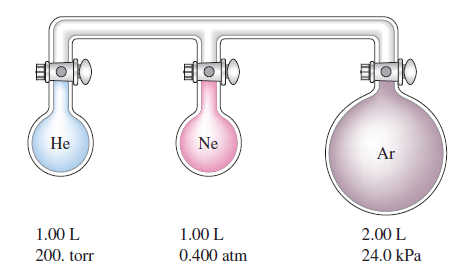# Problem: Consider the three flasks in the diagram below. Assuming the connecting tubes have negligible volume, what is the partial pressure of each gas and the total pressure after all the stopcocks are opened?

###### FREE Expert Solution

Concept: Boyle's Law

From the Ideal Gas Law we get Boyle's Law.        P1V1 = P2V2

Notice that V2 is the total volume after all the stopcocks are opened.         V2 =  1 L + 1 L + 2 L = 4 L

Now we need to find the partial pressures of each gas separately.

83% (360 ratings)###### Problem Details

Consider the three flasks in the diagram below. Assuming the connecting tubes have negligible volume, what is the partial pressure of each gas and the total pressure after all the stopcocks are opened?